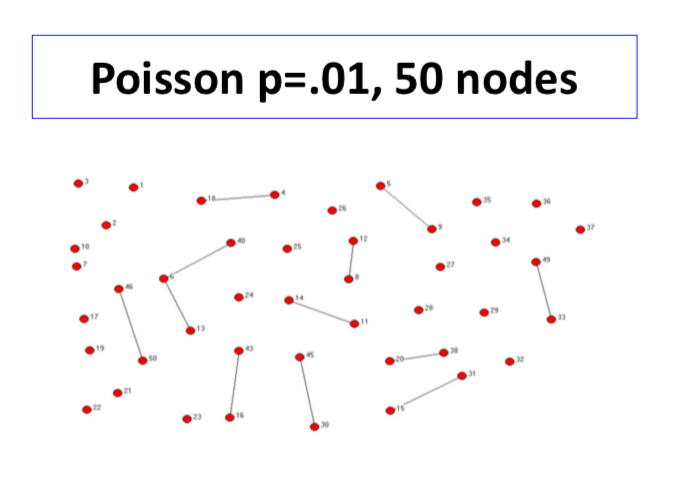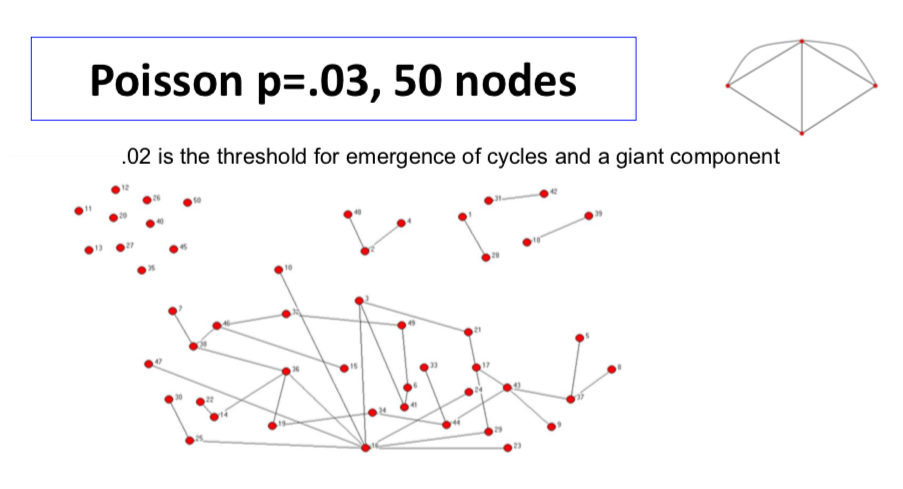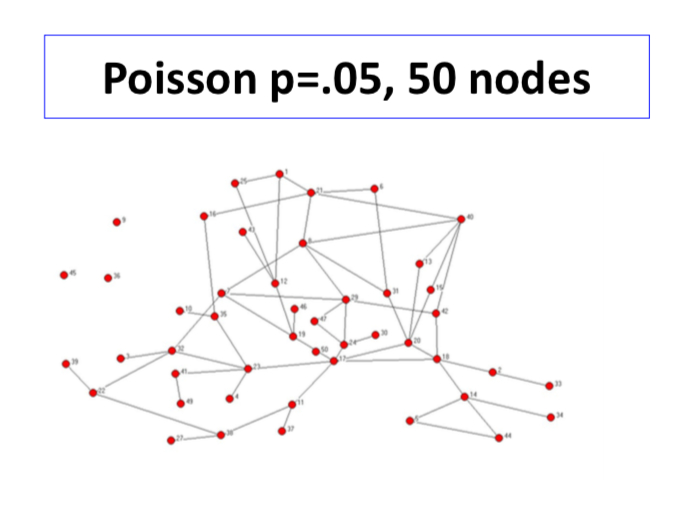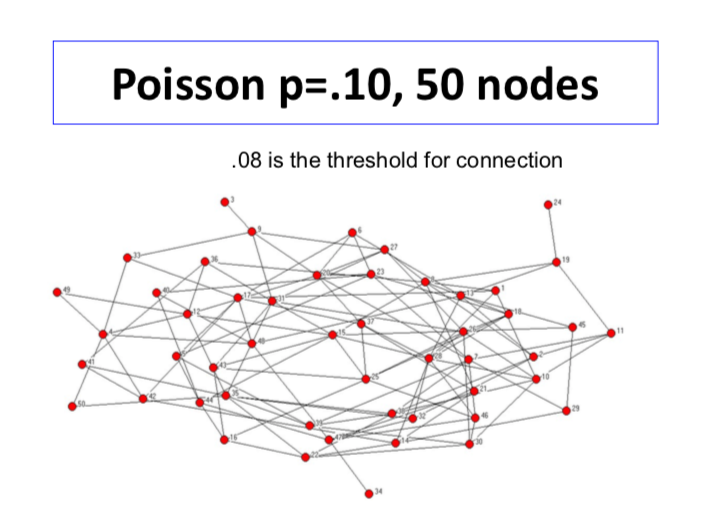# Threshold Function and Phase Transitions¶

• t(n) is a threshold function for a monotone property A(N) if
• Pr[A(N) | p(n)] -> 1 if p(n)/t(n) -> infinity, and
• Pr[A(N) | p(n)] -> 0 if p(n)/t(n) -> 0
• p(n): probability of links
• A phase transition occurs at t(n)

So the idea here is, we'll identify some level of probability that node, that links have to form with. And if you're above that, then the property holds and if you're below that, the property doesn't. So that's a threshold function and we'll say that a phase transition occurs at this threshold, meaning that if your probability is above that, you're getting the property. If not, you don't. So, the the network structure is changing, and either satisfying or not satisfying that property as you cross those thresholds.

# Thresholds for Poisson Random Networks¶

• $1/n^2$ - the network has some links (avg deg 1/n)
• you're not going to get any links with a high probability you won't see any links at all.
• $1/n^{3/2}$ - the network has a component with at least three links (avg deg $1/n^{1/2}$)

• $1/n$ - the network has a cycle, the network has a unique giant component: a component with at least n^a nodes some fixed a<1; (avg deg 1)

• $log(n)/n$ - the network is connected; (avg deg log(n))